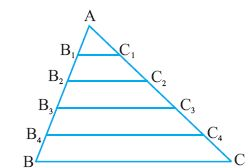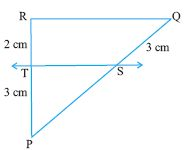Email us to get an instant 20% discount on highly effective K-12 Math & English kwizNET Programs!

#### Online Quiz (WorksheetABCD)

Questions Per Quiz = 2 4 6 8 10

### High School Mathematics - 28.1 Basic Proportionality Theorem or Thale's Theorem

A straight line drawn parallel to a side of a triangle, divides the other two sides proportionally.
Data: Triangle ABC, D is a point on AB and the line DE is parallel to BCConsider triangle ABC and triangle BDE
 Step Statement Reason 1 ang BAC = ang BDE Corresponding angles because DE ll AC 2 ang BCA = ang BED Corresponding angles because DE ll AC 3 ang DBE = ang ABC Common angle 4 triangle ABC lll triangle BDE AAA postulate on similarity 5 AB/BD = BC/BE corresponding sides are proportional 6 (BD+DA)/BD =(BE+EC)/BE Split AB and AC 7 1+AD/BD = 1+CE/BE Simplification 8 AD/BD = CE/BE Subtract 1 from either sides 10 AD/BD= BE/CE Reciprocating

Converse
We shall try to prove the converse of basic proportionality theorem.
Let us consider triangle ABC shown in the figure below.Divide the sides of the triangle AB and AC into any number of equal parts.
By measuring the angles B1 and B we observe that they are equal.
But B1 and B are corresponding angles.
Hence B1C1 ll BC.
Similarly
AB2/B2 B= AC2/C2C = 2/3 and B22C2 ll BC
AB3/B3B =AC3/C3C= 3/2 and B3C3 ll BC
AB4/B4B = AC4/C4C and B4C4 ll BC

Example: In the figure, find the length of PS given that ST ll QR.Let PS = x cm
As ST ll QR
PS/QS = RT/PT
x/3 = 3/2
x = 9/2

 Q 1: M and N are points on the sides PQ and QR of triangle PQR. If PM is 4, QM = 4.5, NR = 4.5 cm. find the length of PN4 cm4.5 cm Q 2: ABC is a triangle. D and E are points on AB and AC. If BD = 4 cms, AD = 8 cms, CE = 9 cms. Find BE.2.5 cms3.5 cms4.5 cms Q 3: A research team wishes to determine the altitude of a mountain as follows: They use a light source at L, mounted on a structure of height 2 meters, to shine a beam of light through the top of a pole P' through the top of the mountain M'. The height of the pole is 20 meters. The distance between the altitude of the mountain and the pole is 1000 meters. The distance between the pole and the laser is 10 meters. We assume that the light source mount, the pole and the altitude of the mountain are in the same plane. Find the altitude h of the mountain. 1000 metres1820 metres500 metres Q 4: ABC is a triangle in which D and E are the points on AC and BC. DE ll AB. CD = 15 cms, AB = 11 cms, DE = 7cms. Find the length of CD9.38.57 Question 5: This question is available to subscribers only! Question 6: This question is available to subscribers only!